Function Repository Resource:

# OsculatingParabola

Get the osculating parabola of a plane curve

Contributed by: Jan Mangaldan
 ResourceFunction["OsculatingParabola"][c,{t,t0},u] gives the parametric equations with parameter u for the osculating parabola of a plane curve c defined with respect to the variable t at t=t0.

## Details

The osculating parabola of a plane curve at a given point is the unique parabola that has third-order contact with the curve at that point.

## Examples

### Basic Examples (2)

Parametric equations for the osculating parabola of a semicubical parabola:

 In:=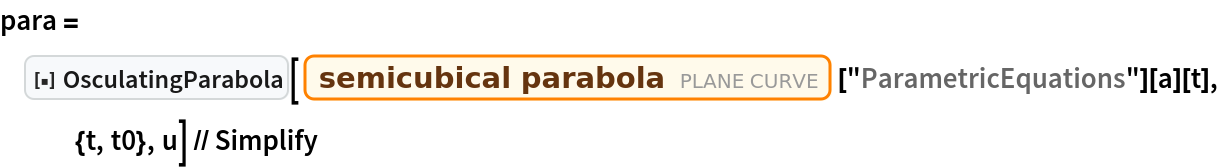Out=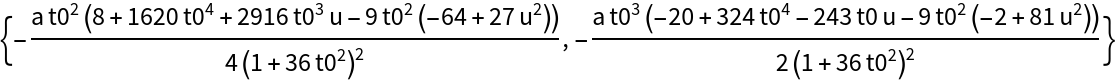Plot the curve and its osculating parabola with the point of contact:

 In:=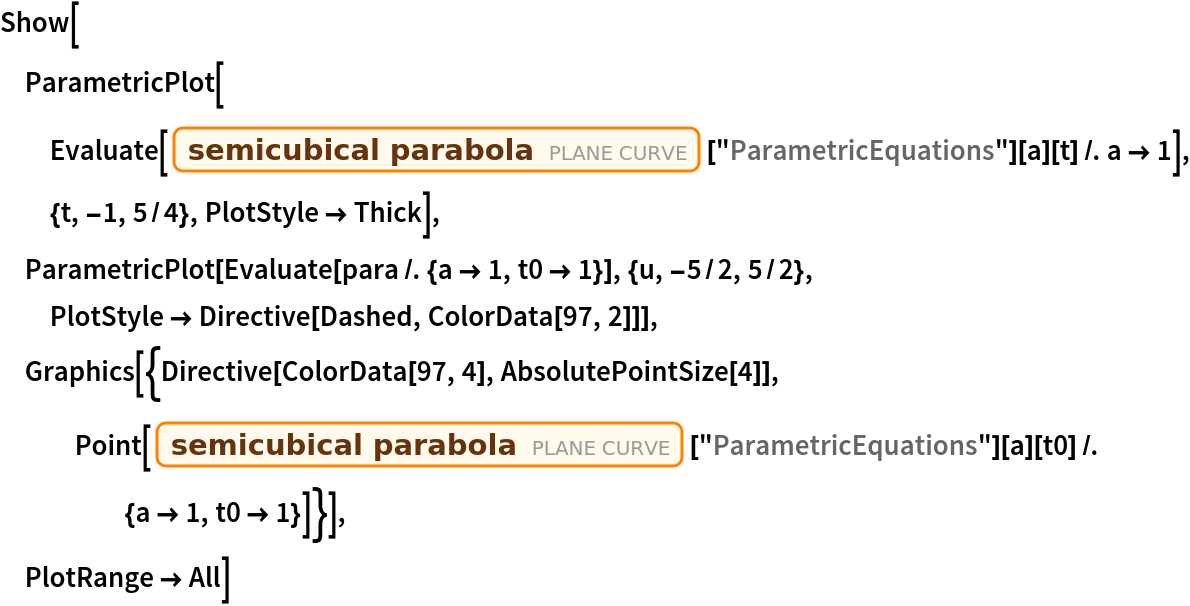Out=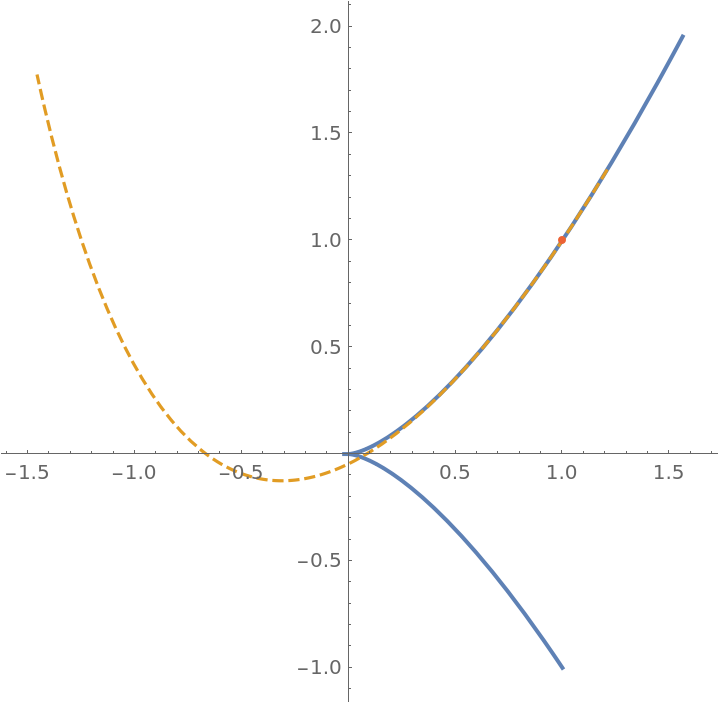Plot a family of osculating parabolas for a cardioid:

 In:=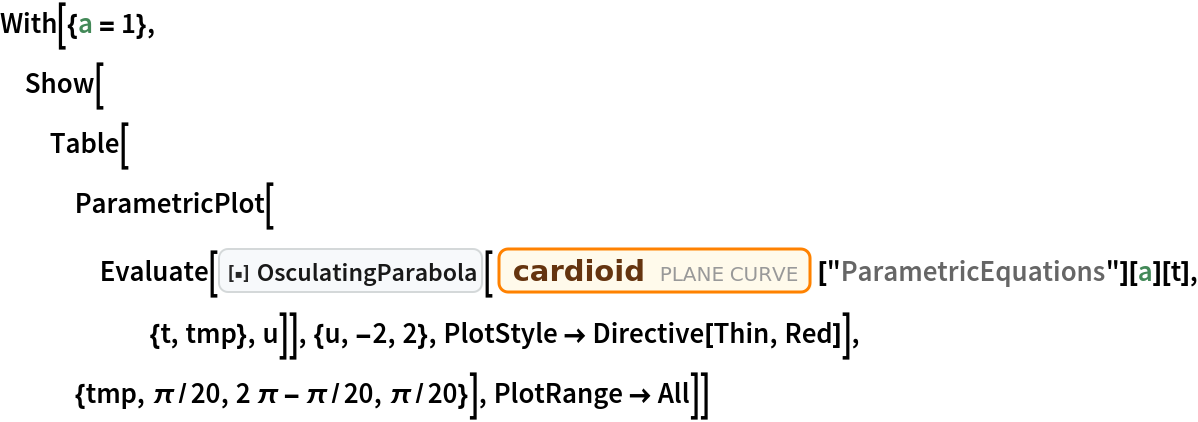Out=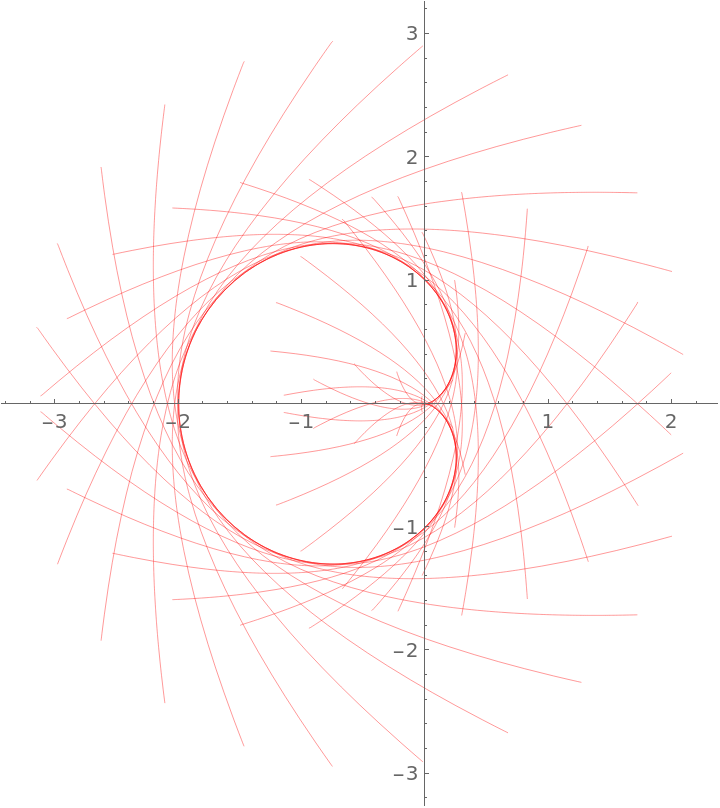### Scope (2)

Generate the implicit Cartesian equation for the osculating parabola of an ellipse at a given point:

 In:=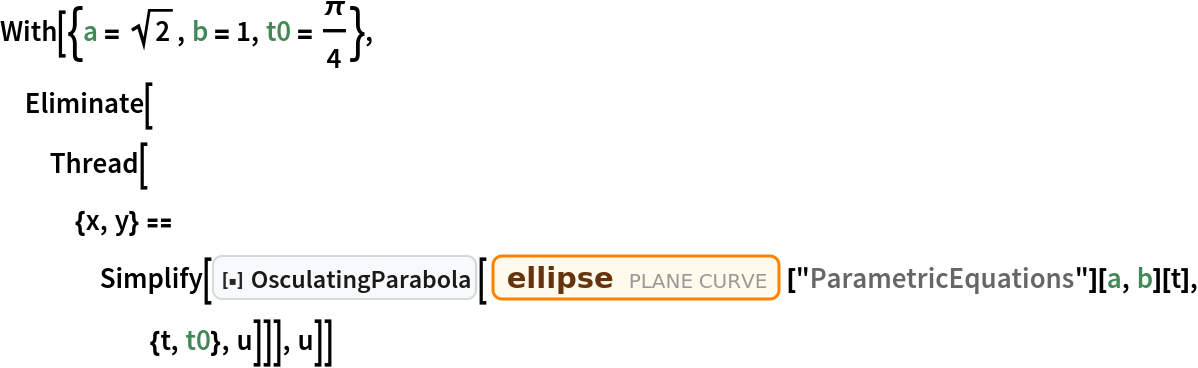Out=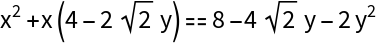Show the ellipse, the osculating parabola, and their point of contact:

 In:=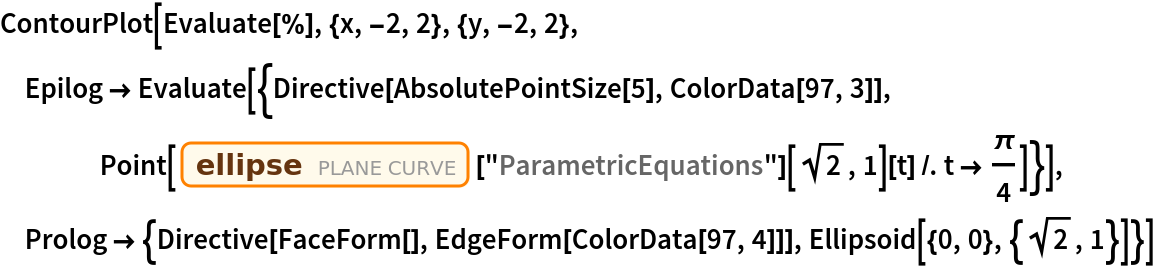Out=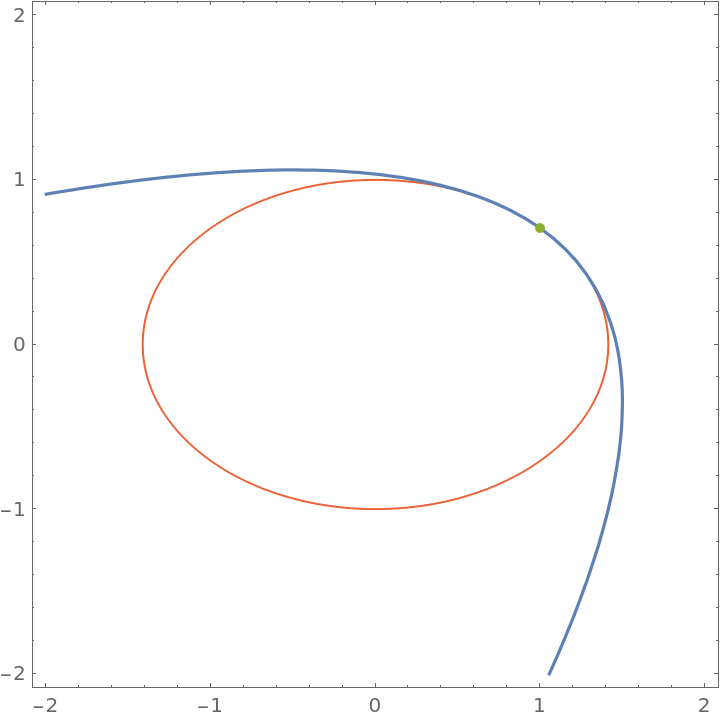## Version History

• 1.0.0 – 15 February 2021